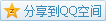# MIT OCW

## 18.02 Multivariable Calculus

The chain rule for a multivariable function $f(x,y,z)$, where $x=x(t),y=y(t),z=z(t)$ is $$\frac{\mathrm{d}f}{\mathrm{d}t}=\frac{\partial f}{\partial x}\frac{\mathrm{d}x}{\mathrm{d}t}+\frac{\partial f}{\partial y}\frac{\mathrm{d}y}{\mathrm{d}t}+\frac{\partial f}{\partial z}\frac{\mathrm{d}z}{\mathrm{d}t}.$$
But now, when the gradient $\nabla f=\left(\frac{\partial f}{\partial x},\frac{\partial f}{\partial y},\frac{\partial f}{\partial z}\right)$ is introduced, the formula has another form $$\frac{\mathrm{d}f}{\mathrm{d}t}=\nabla f\cdot\frac{\mathrm{d}\mathbf{r}}{\mathrm{d}t},$$ where $\mathbf{r}(t)=(x(t),y(t),z(t))$.

#### Relationship with Level Surfaces

The gradient $\nabla f$ is normal to the level surface $F(x,y,z)=c$, also to the tangent plane of the level surface.
Proof Given a function $f(x,y,z)$, and take any curve $\mathbf{r}(t)=(x(t),y(t),z(t))$ on the level surface $f(x,y,z)=c$. According to the chain rule, $$\frac{\mathrm{d}f}{\mathrm{d}t}=\nabla f\cdot\frac{\mathrm{d}\mathbf{r}}{\mathrm{d}t},$$ where $f$ now is on the level surface, so $$\frac{\mathrm{d}f}{\mathrm{d}t}=0,$$ that is $$\nabla f\cdot\frac{\mathrm{d}\mathbf{r}}{\mathrm{d}t}=0.$$

#### Application

##### Tangent Planes

e.g. Solve the tangent plane for $x^2+y^2-z^2=4$ at $(2,1,1)$.
Solution 1 Consider a three-variable function $f(x,y,z)=x^2+y^2-z^2$, then it becomes a level surface $f=4$, since the gradient is normal to the tangent plane of the level surface, so the normal vector of the tangent plane is $$\mathbf{n}=\left(\frac{\partial f}{\partial x},\frac{\partial f}{\partial y},\frac{\partial f}{\partial z}\right)_{(2,1,1)}=(4,2,-2),$$ so we have a equation like $$4x+2y-2z=k,$$ and plug the point into it, we get the tangent plane $$4x+2y-2z=8.$$
Solution 2 Another point of view is at the total differential, near the point that is $$\mathrm{d}f=\frac{\partial f}{\partial x}_{(2,1,1)}\mathrm{d}x+\frac{\partial f}{\partial y}_{(2,1,1)}\mathrm{d}y+\frac{\partial f}{\partial z}_{(2,1,1)}\mathrm{d}z,$$ since we are moving on the level, thus $\mathrm{d}z$ is actually $0$, which gives us $$\frac{\partial f}{\partial x}_{(2,1,1)}\mathrm{d}x+\frac{\partial f}{\partial y}_{(2,1,1)}\mathrm{d}y+\frac{\partial f}{\partial z}_{(2,1,1)}\mathrm{d}z=0,$$ which means $$4(x-x_0)+2(y-y_0)-2(z-z_0)=0,$$ namely $$4(x-2)+2(y-1)-2(z-1).$$

##### Directional Derivative

Sometimes, we care not only the derivative on $\hat\mathbf{i}$ and $\hat\mathbf{j}$ but on some other direction $\hat\mathbf{u}$.
The directional derivative is defined as $$\nabla|_{\hat\mathbf{u}}f=\nabla f\cdot\hat\mathbf{u},$$ it's natural because near some point $$\frac{\mathrm{d}f}{\mathrm{d}s}=\nabla f\cdot\frac{\mathrm{d}r}{\mathrm{d}s},$$ where $s$ is a tiny segment on the direction $\hat\mathbf{u}$, that becomes $$\frac{\mathrm{d}f}{\mathrm{d}s}=\nabla f\cdot\hat\mathbf{u}.$$

#### Geometry

According to the directional derivative, we can write it in a geometric form $$\nabla|_{\hat\mathbf{u}}f=|\nabla f||\hat\mathbf{u}|\cos(\theta),$$ where $\theta$ is the angle between $\nabla f$ and $\hat\mathbf{u}$.

• When $\theta=0$, the directional derivative is maximal, so the function increases fastest in the direction of $\nabla f$;
• When $\theta=\pi$, the directional derivative is minimal, so the function decreases fastest in the opposite direction of $\nabla f$;
• When $\theta=\frac\pi2$, the directional derivative is $0$, so the function does not change and stay on a level surface.
So, the gradient points at the direction where the function has a max rate of change.